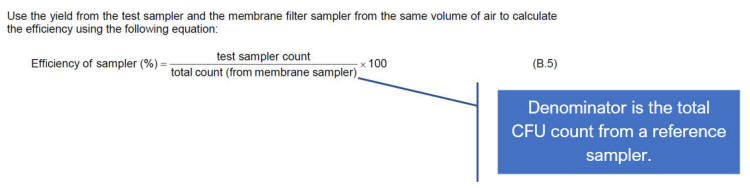# Theoretical d50 Calculator

The following is a calculator for BS EN 17141:2020 compliance for microbial air samplers.

 Flow Rate (LPM) # of inlets Diameter of Inlets (mm)
##### What is the d50?

The d50 is the particle size (µm) at which there's a 50% physical collection efficiency.

Due to mass, particle sizes larger than this d50 value generally have a higher than 50% collection efficiency.  And, particles sizes lower that the d50 value have less than a 50% probability of being collected.

There is both a theoretical d50 value based on a calculated fluid dynamics math equation, and an experimental d50 value that provides an instruments actual physical collection efficiency. The experimental d50 is vastly more important than the calculated d50.

##### Background

A problem was introduced with the ratification of ISO 14698-1:2003, which attempted to provide a method of validating a microbial air sampler.  The 'Sampling Efficiency' equation (B.5) was provided in Annex B, which is an informative section of the standard.The method has one main fault. It fails to report the number of particles not collected by the air sampler. (Yao, 2006) Also, it is well known that Physical and Biological Collection efficiency of the numerous samplers in manufacture today can vary greatly. Subsequently, comparing one sampler against a low efficiency sampler may yield over inflated results and false sense of assurance. The equation is not so much a 'Sampling Efficiency' as it's a Comparative Efficiency.

An article was written in 2006, which provides a calculated fluid dynamics (CDF) equation to estimate the d50 value.  That is, the particle size at which there's a 50% collection efficiency (Yao, 2006).   An important element of this article is the theoretical d50 was compared to an experimental d50 using a particle counter. It was frequently found that the experimental d50 was much larger than the theoretical d50. Root cause for this variance is a design error.